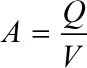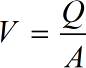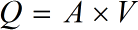Request a Tool

Flow Rate Calculator

The online Flow Rate Calculator is used to calculate the flow rate, flow area and flow velocity of the fluid

Flow Area
0

Formula• A = Flow Area
• Q = Flow Rate
• V = Flow Velocity

Defination / Uses

The amount of fluid that flows in a particular amount of time is referred to as flow rate. The flow rate, flow area, and flow velocity of a fluid are calculated using the online advanced Flow Rate Calculator. The flow rate calculator is used to calculate the fluid flow rate through a given substance or container.

Flow Velocity
0

Formula• V = Flow Velocity
• A = Flow Area
• Q = Flow Rate

Defination / Uses

The amount of fluid that flows in a particular amount of time is referred to as flow rate. The flow rate, flow area, and flow velocity of a fluid are calculated using the online advanced Flow Rate Calculator. The flow rate calculator is used to calculate the fluid flow rate through a given substance or container.

Flow Rate
0

Formula• Q = Flow Rate
• V = Flow Velocity
• A = Flow Area

Defination / Uses

The amount of fluid that flows in a particular amount of time is referred to as flow rate. The flow rate, flow area, and flow velocity of a fluid are calculated using the online advanced Flow Rate Calculator. The flow rate calculator is used to calculate the fluid flow rate through a given substance or container.

How calculte the flow rate?

There are only two basic steps.

• First select the option from dropdown. What you need to calculate.
• Then add the other values in their corresponding fields.

Thats it! You will get your answer in less than a second. Use upper given formula in case of manual calculation.

Flow rate and velocity are two physical characteristics that are related but not the same. Consider the flow rate of a river to make the distinction evident. Our flow rate calculator, believe it or not, is useful not only in fluid mechanics but also in everyday concerns. It will assist you not only in determining the flow rate of a garden hose or shower head, but also in determining the amount of blood your heart pumps each minute (cardiac output). It can also be used to calculate the velocity of a pipe. This explains what is flow rate and how to calculate it. Be cautious, as the word "flow rate" may be misleading in and of itself! Fortunately, we've included two flow rate formulas, so you'll be covered in either circumstance. For quick calculation use our weetools. No sign-up, registration OR captcha is required to use this tool.